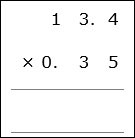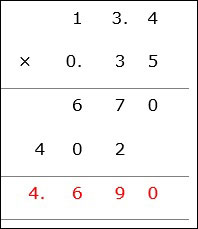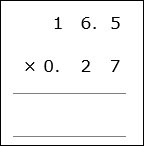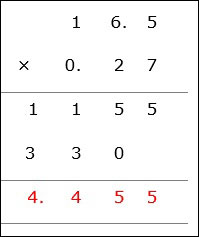# Decimal Multiplication: Problem Type 1

Decimal multiplication is done in a similar way as whole number multiplication.

Rules for Decimal Multiplication

• First we multiply the decimal numbers as if they were whole numbers by ignoring the decimal points.

• Here the decimal points are not lined up vertically as in addition and subtraction. Here the digits are lined up to the right.

• The number of digits after the decimal point in both the numbers are counted and added.

• In the final product, a decimal point is placed before that many digits from the right.

Multiply 13.4 × 0.35

### Solution

Step 1:

13.4 × 0.35

We line up the digits and not the decimal pointsWe now multiply the numbers by ignoring the decimal points and assuming as if they were whole numbers.

Step 2:

13.4 has one decimal place. 0.35 has two decimal places. So the product of these numbers has three decimal places.Multiply 16.5 × 0.27

### Solution

Step 1:

16.5 × 0.27

We line up the digits and not the decimal pointsWe now multiply the numbers by ignoring the decimal points and assuming as if they were whole numbers.

Step 2:

16.5 has one decimal place. 0.27 has two decimal places. So the product of these numbers has three decimal places.